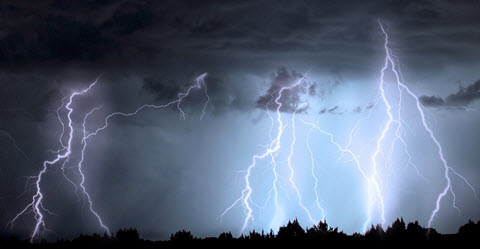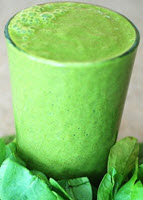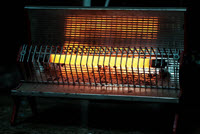# PowerPower is energy flowing! It is measured as energy per unit of time

• Energy is in Joules (J)
• Power is in Joules per second (J/s) which has the special unit of Watts (W)

1 Watt = 1 Joule of energy per second

1 W = 1 J/s

## Power FormulasNo, not that type of Power Formula!

Power is the rate of energy per time:

P = Energy Time

### Example: 3000 J of energy is used in 20 seconds, what is the power?

P = 3000 J 20 s = 150 W

We can also change the formula this way:

Energy is also Force × Distance:P = Force × Distance Time
Distance/Time is Velocity, so:P = Force × Velocity### Example: These guys pull with a combined force of 4000 N, and manage a velocity of 0.4 m/s, what power are they using?

P = 4000 N x 0.4 m/s
= 1600 N m/s
= 1600 W

Hang on! Is N m/s (Newton meter per second) really the same as W (Watt)?

In fact all these are the same:

 Power is: Start with: N m/s A Newton is kg m/s2: kg m/s2 m/s Simplify: kg m2/s3 A Joule is kg m2/s2: J/s A Watt is J/s: W

## Horsepower

1 horsepower (hp) is 745.7 W (or 746 W for electrical motors)

### Example: Sam's car has a 200 hp engine. What is that in watts?

200 × 745.7 = 149,140 W = 149 kW (to nearest kW)

## What is a kiloWatt Hour?

1 kWh is the Energy used when the Power is 1 kW for 1 hour.### This is a little 1 kW radiator. How much energy does it use in an hour?

• 1 kW is 1000 Watts which is 1000 J/s
• There are 3600 seconds in an hour

So in an hour it uses:

1000 J/s × 3600 s = 3,600,000 J = 3.6 MJ

And so 1 kWh (kiloWatt hour)  is 3.6 MJ of Energy

## Electric Power

Electric Power has its own special formula, but it still end up as Watts:17609, 17611, 17613, 17615, 17619, 17616, 17618, 17622, 17620, 17628